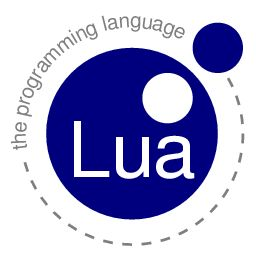# Lua Primer for the ImpatientLua is one of the scripting languages supported by GLSL Hacker. Here is a quick introduction to the essential notions and syntax of Lua programming language. All following notions are general and are not specific to GLSL Hacker. Thanks to this primer, you will be able to quickly tweak and hack GLSL Hacker demos. GLSL Hacker 0.5.0 uses Lua 5.2.2.

The reference manual of Lua 5.2 can be found HERE and the first edition of the PIL book (Programming in Lua) is available online HERE.

Single line comment: —

```-- this is a single line comment in Lua
```

Multi-line comment: –[[ ]]–

```--[[
this is a
multi-line
comment in Lua
--]]
```

The nice thing with multi-line comments is that you can toogle comment ON/OFF by adding a – at the beginning of the comment.

Comment disabled:

```---[[
ThisFunctionIsNotCommented()
--]]
```

Comment enabled:

```--[[
ThisFunctionIsCommented()
--]]
```

## 2 – Variables

Variables in Lua are typeless, case sensitive and by default the scope of a variable is global.

```num_vertices = 0
num_vertices = 1000
mesh_name = "my_kool_mesh"
```

Lua supports also local variables. Local variables are faster but their scope are limited to the block where they have been declared.

```local i=0
```

In Lua, all numbers are double precision floating point numbers.

To concatenate strings, just use the .. operator:

```local space = " "
app_name = "GLSL" .. space .. "Hacker"
```

## 3 – Functions

Functions in Lua can take multiple arguments and can return multiple results.

```function myKoolFunc(a, b, c)
-- Do something useful with a, b and c:
local sum = a+b+c
local avg= sum/3
return sum, avg
end

x, y = myKoolFunc(1, 2, 3)
```

## 4 – Tables

The table is one of key elements of Lua. The table is the funcdamental element for creating data structures in Lua.

You can create an empty table like this:

```my_table = {}
```

You can store whatever you want in a table: numbers, strings, tables, functions…

You can create and initialize an array with fields:

```color = {r=0, g=0.25, b=1}
```

You can dynamically add new fields to a table:

```my_table = element1
my_table = element2
my_table = "a string"
my_table.the_func = myKoolFunc
local color = {r=0, g=0, b=0}
my_table.the_color = color
my_table.the_color.avg = (color.r + color.g + color.b) / 3
```

The first element of a table is indexed by 1 and not 0 like in C.

## 5 – Operators

Comparison:

• equal: (a == b)
• not equal: (a ~= b)
• greater than: (a > b)
• greater than or equal: (a >= b)
• lesser than: (a < b)
• lesser than or equal: (a <= b)

Logical:

• and: (a and b)
• or: (a or b)

## 6 – Control Structures

Conditional tests:

```if ((a > x) and (a < y)) then
-- do something
elseif (a == y) then
-- do something
else
-- do something
end
```

Loops

for:

```local i
for i=0, 10 do
-- do something
end
```

while:

```local i=0
while (i < 10) do
-- do something
i=i+1
end
```

The break keyword can be used to jump out from a loop:

```local i=0
while (i < 10) do
-- do something
if (i==5) then
break
end
i=i+1
end
```

## 7 - Built-in functions

Lua comes with a set of built-in functions exposed by the Lua standard libraries. Here are some of the most important libraries:

### Mathematical library

Mathematical functions are accessible via the math library.

```sin_alpha = math.sin(alpha)
cos_alpha = math.cos(alpha)
```

Random numbers: math.random() returns a number in the [0.0 ; 1.0] interval:

```x = math.random()
```

When math.random is called with two arguments a and b, it returns a number (an integer) in the [a ; b] interval:

```-- possible values for y: 2, 3, 4 and 5.
y = math.random(2, 5)
```

### String Library

The string library allows to play with strings.

An useful function is the format function that creates a formated string like C printf().

```vertices = 1000
faces = 3000
str = string.format("Vertices=%d, Faces=%d", vertices, faces)
```